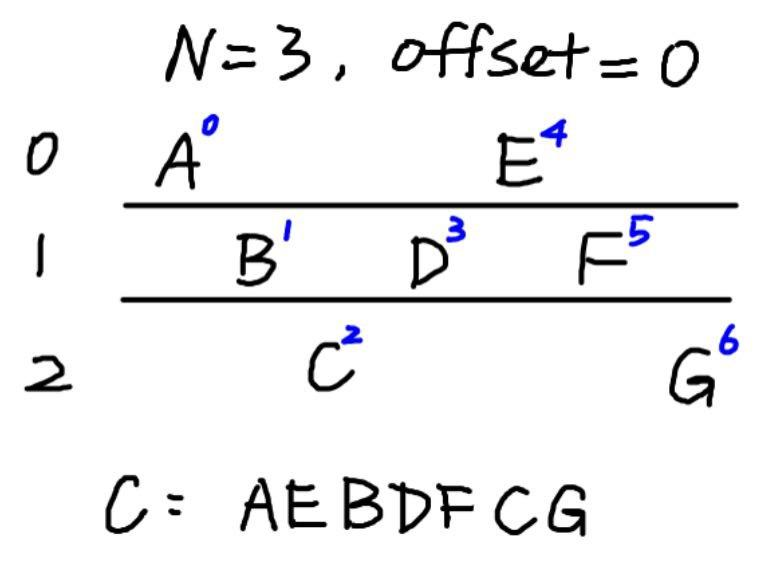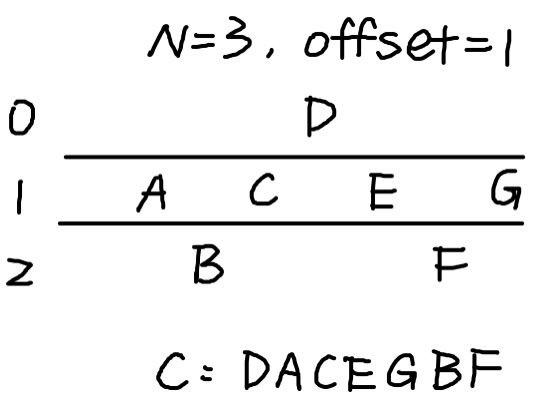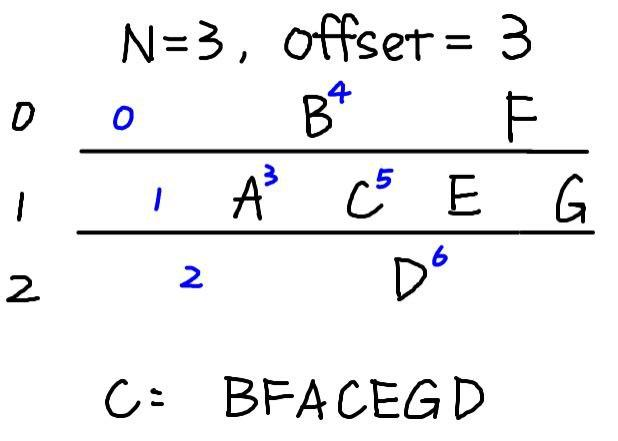# He Thrusts His Fists Against the Post

## 题目¶

{ea-vetgaytereoreh-na}fan--wshbestpasslds-s-hm

## 解题思路¶

• 由花括号和 flag 相关字母推测是置换密码，由此想到「栅栏密码」
• 因为 { 在首位，加密的偏移量显然不为 $$0$$
• 枚举分组长度和偏移量，当分组长度为 $$4$$，偏移量为 $$2$$ 时，解出 Flag：flag{and-yet-swears-he-observes-the-phantasms}

### 偏移量 Offset¶• 明文消息为 $$M$$，加密时，栅栏密码分组长度 $$N$$ 可以视为 $$N$$ 行，偏移量 $$x$$ 表示从第 $$x$$ 行开始输入字符• 可以扩展到 $$N \le x < 2 \times (N - 1)$$（当 $$x \ge 2 \times (N - 1)$$ 时，与 $$N \le x < 2 \times (N - 1)$$ 包含的情况一致），表示从第 $$x$$ 个位置（按照没有偏移量时的输入位置）开始输入字符## 参考资料¶

Rail Fence, Zig-Zag - online encoder / decoder

Contributors: YanhuiJessica
Pageviews: